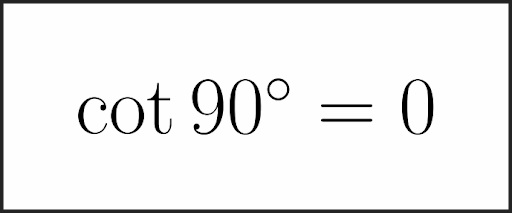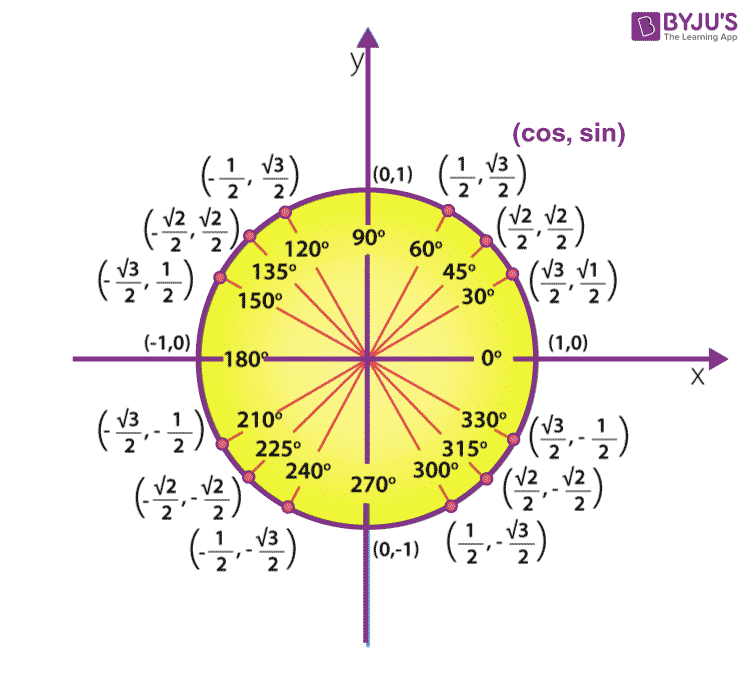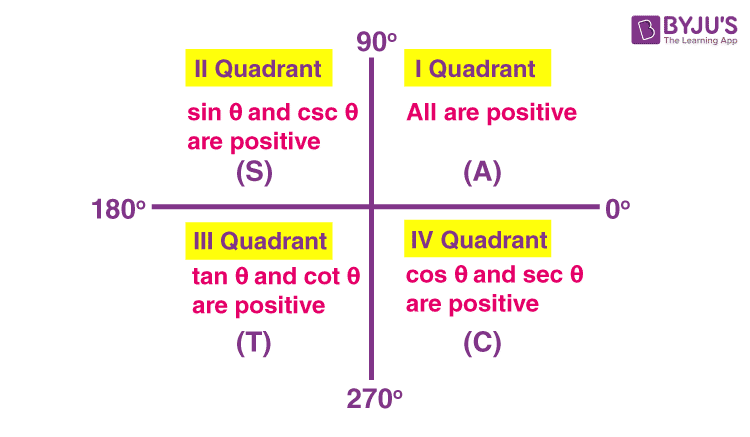# Cot 90

In trigonometry, the value of cot 90 (in degrees) is equal to 0. The related formulas based on this value can be derived using trigonometry ratios of complementary angles. The trigonometric Table of sin, cos, tan, cosec, sec and cot for the standard angles from 0° to 360° is used to solve many problems in maths. We can apply different formulas in trigonometry to get the required values for trigonometric functions. In this article, you will learn what the cot 90 value is and find the value of cot 90° with solved examples based on the cot 90 value.

## Cot 90 Value

Cot 90 value (in degrees) is given as:What is Cot 90 degrees in radians?

In a circular system, cot 90° can be written as cot π/2.

As we know the cot can be expressed as cos over sin. This relation helps in deriving the cot 90 value from the unit circle.

For a unit circle, which has a radius equal to 1, we can derive the tangent values of all the degrees. With the help of a unit circle drawn on the XY plane, we can find out all the trigonometric ratios and values.In the above figure,

sin 90° = 1 and cos 90° = 0

Now,

cot 90° = cos 90°/sin 90° = 0/1 = 0

Therefore, the value of Cot 90 degrees is equal to zero.

Also, get the trigonometric functions calculator here to find the values for all the angles.

## Cot 90 Degrees

In this section, you will learn how to derive the value of cot 90 degrees value.

We know that,

sin 90° = 1, cos 90° = 0 and tan 90° = sin 90°/cos 90° = 1/0

Now,

cot 90° = 1/tan 90°

= 1/(1/0)

= 1 × (0/1)

= 0

The below figure shows the sign of trigonometric functions in different quadrants.This helps in deriving the complementary angles of various trigonometry functions in maths.

### Cot 90 – Theta

Let us derive the formula for cot 90 minus theta or cot 90 – theta, i.e. cot(90° – θ).

cot(90° – θ) = cot[1 × 90° – θ]

Here, 90° is multiplied by an odd number 1. So the trigonometric function will change to its reciprocal function. That means, in the next step we should write “tan”.

Also, 90° – θ lies in the first quadrant, where tan is positive (all the six functions are positive in this quadrant).

Thus, cot(90° – θ) = tan θ

Similarly, cot(90° – x) = tan x

And

cot(90° – a) = tan a

All these formulas can also be expressed as:

cot(π/2 – θ) = tan θ

cot(π/2 – x) = tan x

cot(π/2 – a) = tan a

### Cot 90 Plus Theta

Let us derive the formula for cot 90 + theta, i.e. cot(90° + θ).

cot(90° + θ) = cot[1 × 90° + θ]

Here, 90° is multiplied by an odd number 1. So the trigonometric function will change to its reciprocal function. That means, in the next step we should write “tan”.

Also, 90° + θ lies in the second quadrant, where tan is not positive (only sine and cosecant are positive in this quadrant).

Thus, cot(90° + θ) = -tan θ

Similarly, cot(90° + x) = -tan x

And

cot(90° + a) = -tan a

All these formulas can also be expressed as:

cot(π/2 + θ) = -tan θ

cot(π/2 + x) = -tan x

cot(π/2 + a) = -tan a

Let us recall the complementary angles formulas in trigonometry so that we can easily understand this method of derivation formulas based on cot function.

• sin (90° – A) = cos A
• cos (90° – A) = sin A
• tan (90° – A) = cot A
• cot (90° – A) = tan A
• sec (90° – A) = cosec A
• cosec (90° – A) = sec A
• sin(90° + A) = cos A
• cos(90° + A) = -sin A
• tan(90° + A) = -cot A
• cot((90° + A) = -tan A
• sec(90° + A) = -cosec A
• cosec(90° + A) = sec A

### Where is Cot Undefined

The value of cot is undefined for some specific angles. This can be understood from the table of trigonometry ratios given below.

 Trigonometry Ratios Table Angles (In Degrees) 0° 30° 45° 60° 90° 180° 270° 360° Angles (In Radians) 0° π/6 π/4 π/3 π/2 π 3π/2 2π sin 0 1/2 1/√2 √3/2 1 0 -1 0 cos 1 √3/2 1/√2 1/2 0 -1 0 1 tan 0 1/√3 1 √3 ∞ 0 ∞ 0 cot ∞ √3 1 1/√3 0 ∞ 0 ∞ cosec ∞ 2 √2 2/√3 1 ∞ -1 ∞ sec 1 2/√3 √2 2 ∞ -1 ∞ 1

Thus, cot value is undefined for 0°, 180° and 360°. These values are written as undefined or infinity (∞) as the ratio of cos and sin at these angles is equal to 1/0.

Visit www.byjus.com to get the value of cot for various angles. Also, download BYJU’S – The Learning App to get more information on trigonometry.

## Frequently Asked Questions on Cot 90

### What is the COT of 90?

The cot 90 can be written as cot 90 degrees, i.e. cot 90° and is equal to 0.

### Is cot 90 undefined?

No, the value of cot 90 is defined and its value is 0.

### Why is COT 90 defined?

The value of cot 90 is defined since the ratio of cos 90 degrees to sin 90 degrees is defined to a finite value. That means, sin 90° = 1, cos 90° = 0 and cot 90° = cos 90°/sin 90° = 0/1 = 0.

### What is the value of cot 90 plus Theta?

The value of cot 90 plus theta can be written as cot(90° + θ) and its value is -tan θ.Therefore, cot(90° + θ) = -tan θ.

### What is COT 90 minus theta?

The value of cot 90 minus theta can be written as cot(90° – θ) and its value is tan θ. Therefore, cot(90° – θ) = tan θ.